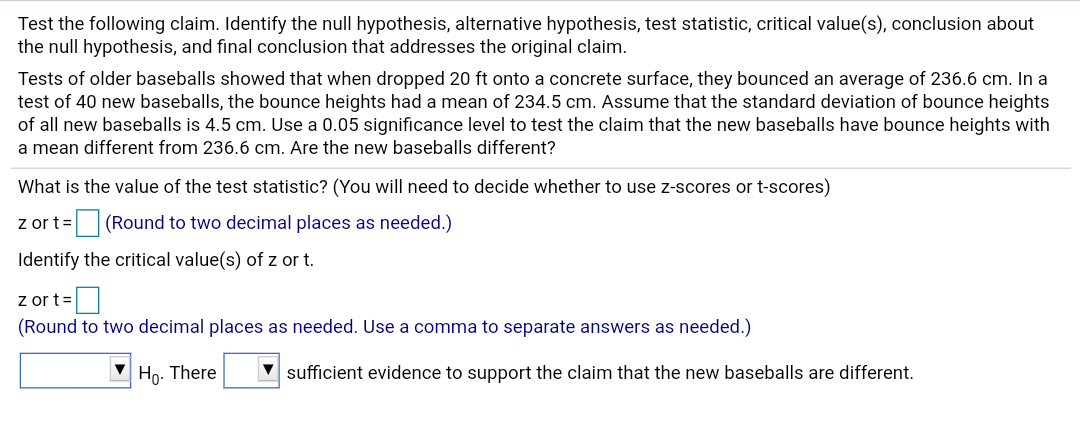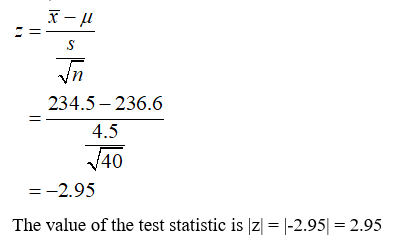# Test the following claim. Identify the null hypothesis, alternative hypothesis, test statistic, critical value(s), conclusion aboutthe null hypothesis, and final conclusion that addresses the original claim.Tests of older baseballs showed that when dropped 20 ft onto a concrete surface, they bounced an average of 236.6 cm. In atest of 40 new baseballs, the bounce heights had a mean of 234.5 cm. Assume that the standard deviation of bounce heightsof all new baseballs is 4.5 cm. Use a 0.05 significance level to test the claim that the new baseballs have bounce heights witha mean different from 236.6 cm. Are the new baseballs different?What is the value of the test statistic? (You will need todecide whether to use z-scores or t-scores)z or t= (Round to two decimal places as needed.)Identify the critical value(s) of z or t.z or t=(Round to two decimal places as needed. Use a comma to separate answers as needed.)V Ho. ThereV sufficient evidence to support the claim that the new baseballs are different.

Question
2 viewshelp_outlineImage TranscriptioncloseTest the following claim. Identify the null hypothesis, alternative hypothesis, test statistic, critical value(s), conclusion about the null hypothesis, and final conclusion that addresses the original claim. Tests of older baseballs showed that when dropped 20 ft onto a concrete surface, they bounced an average of 236.6 cm. In a test of 40 new baseballs, the bounce heights had a mean of 234.5 cm. Assume that the standard deviation of bounce heights of all new baseballs is 4.5 cm. Use a 0.05 significance level to test the claim that the new baseballs have bounce heights with a mean different from 236.6 cm. Are the new baseballs different? What is the value of the test statistic? (You will need to decide whether to use z-scores or t-scores) z or t= (Round to two decimal places as needed.) Identify the critical value(s) of z or t. z or t= (Round to two decimal places as needed. Use a comma to separate answers as needed.) V Ho. There V sufficient evidence to support the claim that the new baseballs are different. fullscreen
check_circle

Step 1

From the provided information,

Sample size (n) = 40

The mean of bounce height () = 234.5 cm

Standard deviation (s) = 4.5 cm

The hypotheses can be constructed as:

Null hypothesis: H0:µ=236.6 cm

Alternative hypothesis: H0:µ≠236.6 cm

Here µ is the population mean. The test is two tailed.

Step 2

Since the sample size is greater than 30 so the z test can be used. When n > 30 than σ can be replaced by s.

Now, the test statistic can be obtained as:...

### Want to see the full answer?

See Solution

#### Want to see this answer and more?

Solutions are written by subject experts who are available 24/7. Questions are typically answered within 1 hour.*

See Solution
*Response times may vary by subject and question.
Tagged in

### Statistics